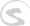Documents
Writing Docs Gigedit SFZ Instrument Scripts NKSP Language NKSP Reference

# log10()

Calculates and returns logarithm to base 10 of the passed `number`.

### Function Prototype

`log10(number) `

### Arguments

Argument Name Data Type Description
`number` Real Number Input value.
[required]

### Return Value

Data Type Description
Real Number Logarithm to base 10 of originally passed `number`.

### Remarks

This functions accepts any standard unit and finalness for its argument `number`. The return value's standard unit and finalness is identical to the passed `number`'s one.

### Examples

None yet.

`log()`, `log2()`, `round()`, `sqrt()`, `exp()`, `pow()`, `sin()`